# Cohomology ring

A ring the additive group of which is the graded cohomology group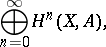whereis a chain complex,is a coefficient group and the multiplication is defined by the linear set of mappingsfor all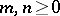, which are the inner cohomology multiplications (cup products). The cohomology ring turns out to be equipped with the structure of a graded ring.

For the existence of the mappings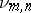it is enough to have a set of mappingssatisfying certain additional properties, and a mapping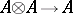, that is, a multiplication in the coefficient group(see ). Theinduce mappings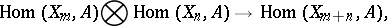which in their turn induce mappingsin cohomology.

In particular, a ring structure is defined on the graded group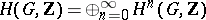, whereis a group and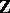is the ring of integers with a trivial-action. The corresponding mappingscoincide with the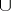-product. This is an associative ring with identity, and for homogeneous elements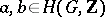of degrees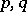respectively,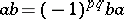.

Analogously, the-product defines a ring structure on the group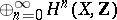, where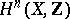is the-dimensional singular cohomology group of a topological spacewith coefficients in.